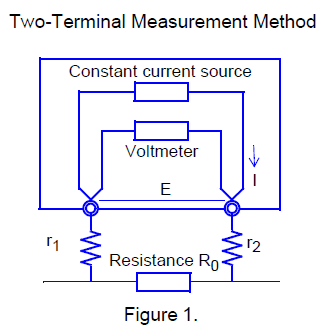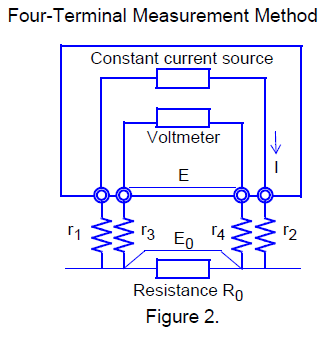# 4-terminal resistance measurement method (4-terminal method)

## QWhat is the measuring principle of the 4-terminal method? (Resistance Meter)

A

Hioki resistance meters use the "4-terminal method" to minimise measurement errors when measuring small resistances.
Most testers use the 2-terminal method (see Figure 1), which means that the resistance of the measuring lead itself is added to the resistance to be measured, causing an error. On the other hand, the 4-terminal measurement (see Figure 2) consists of a current source terminal for supplying a constant current and a voltage detector terminal for detecting a voltage drop. A voltmeter with a high input impedance is connected to the voltage detection terminal side connected to the resistor under test. As very little current flows through this lead, it can be measured accurately without being affected by the resistance or contact resistance of the measurement lead.

<Figure 1>
Measurement current I flows through test object resistance R0 as well as lead resistances r1 and r2.The voltage to be measured is obtained by E = I (r1 + R0 + r2), which includes lead resistances r1 and r2.

<Figure 2>
Current I flows from r2 through DUT resistance R0 and through r1. The high input impedance of the voltmeter allows only negligible current flow through r3 and r4. So the voltage drop across r3 and r4 is practically nil, and voltage E across the measurement terminals and voltage E0 across test object resistance R0 are essentially equal, allowing test object resistance to be measured without being affected by r1 to r4.

- Contact Resistance
Contact resistance is a resistance that occurs on a contact surface due to roughness, oxide film, oil, rust, dust and other debris.Depending on the environmental conditions, contact resistance can range from a few ohms to several tens of ohms, and the value is often unstable. This is one of the reasons why it is difficult to measure low resistance using a two-terminal measurement method using a tester.

••••